# RS Aggarwal Solutions for Class 8 Chapter 3 - Square and Square Roots Exercise 3A

The pdf of RS Aggarwal Solutions for the Exercise 3A of Class 8 Maths Chapter 3, Squares and Square Roots are available here. The questions present in the Exercise 3A have been solved by BYJU’S experts in Maths, and this will help the students in solving the questions without any error. As this is the first exercise, this will help the students to understand the basic concepts of the perfect square as well as the prime factorisation method. In this exercise, there are 6 questions and each having sub-questions. By practising the RS Aggarwal Solutions for class 8, students will be able to grasp the concepts perfectly. Hence, students whose aim is to score high in Maths examinations are advised to go through RS Aggarwal Solutions for Class 8.

## Download PDF of RS Aggarwal Solutions for Class 8 Chapter 3 – Squares and Square Roots – Exercise 3A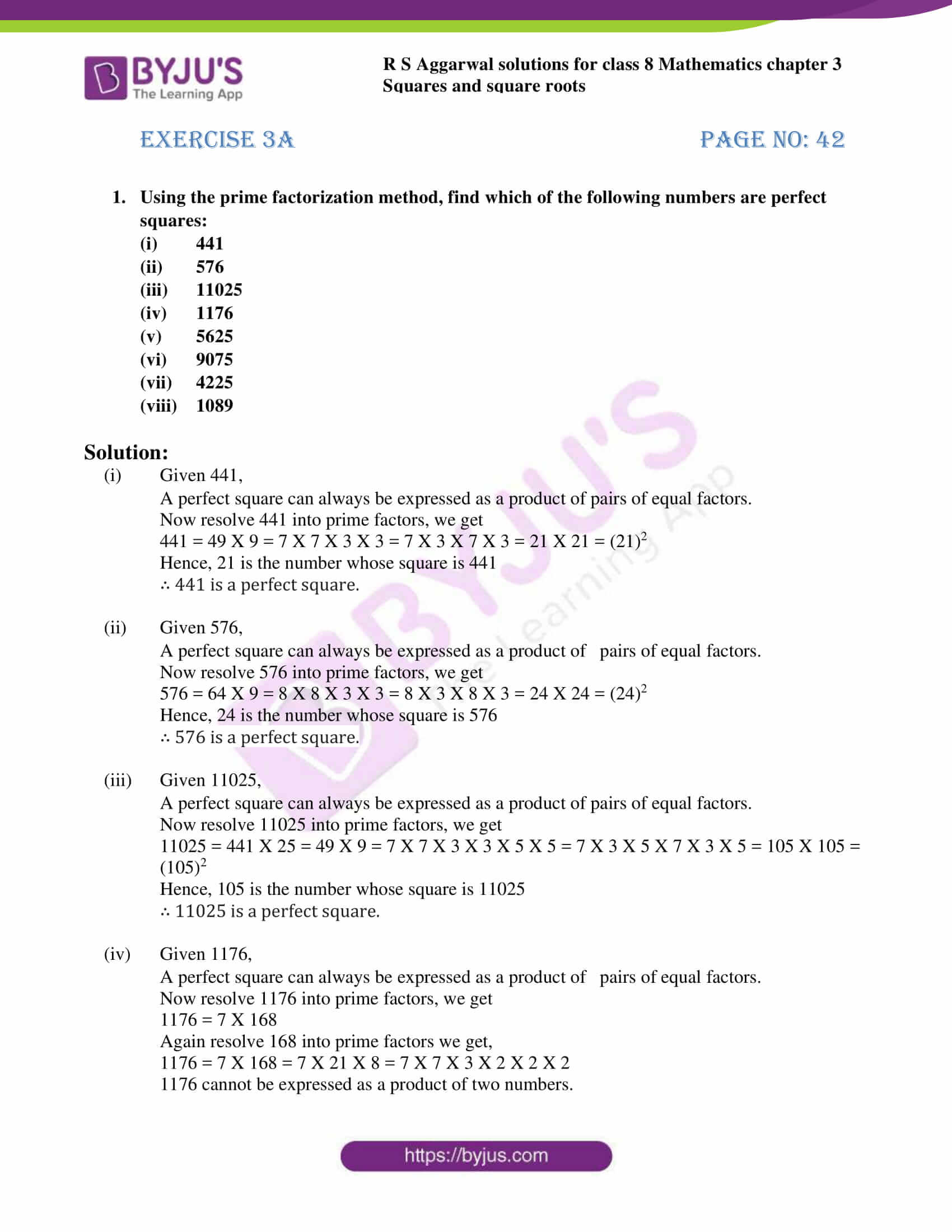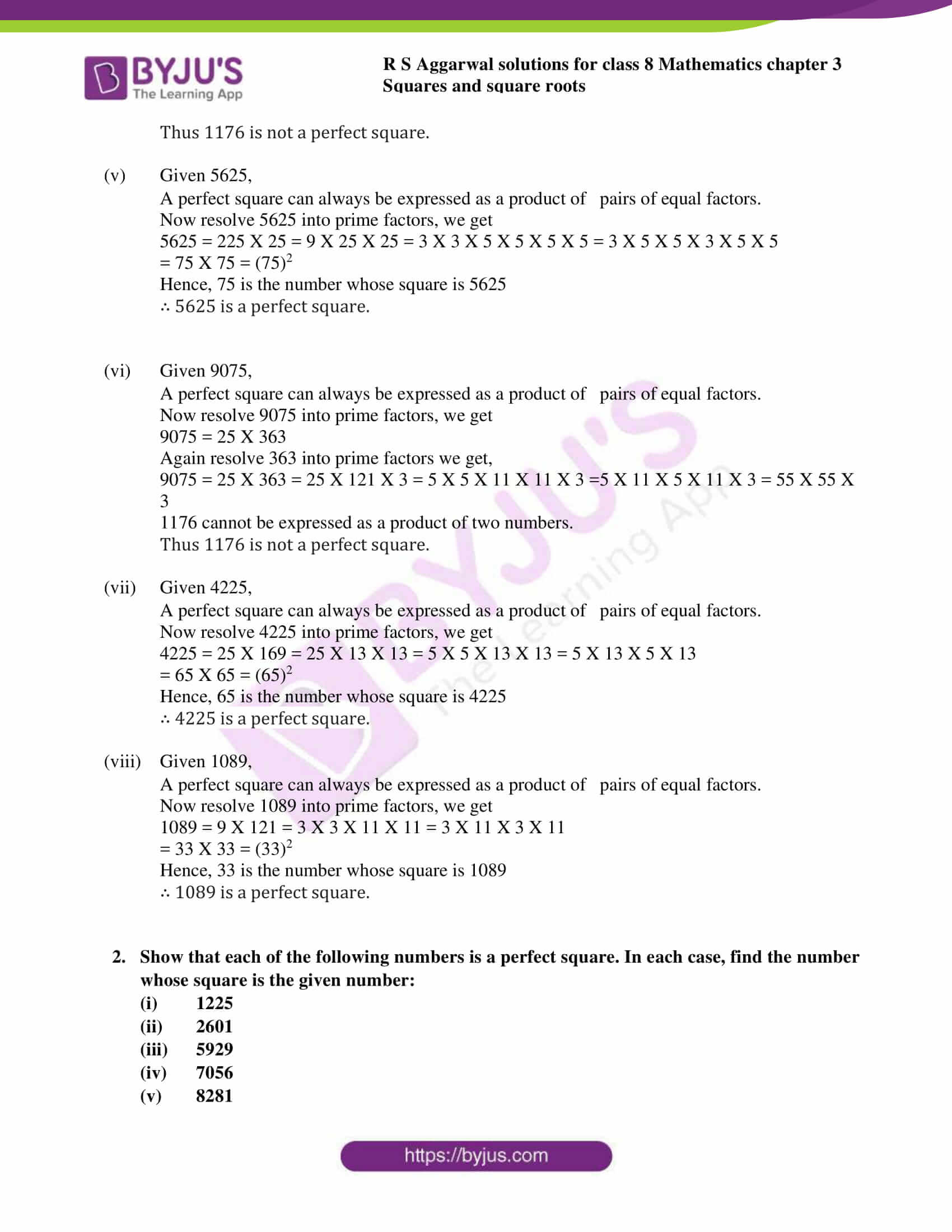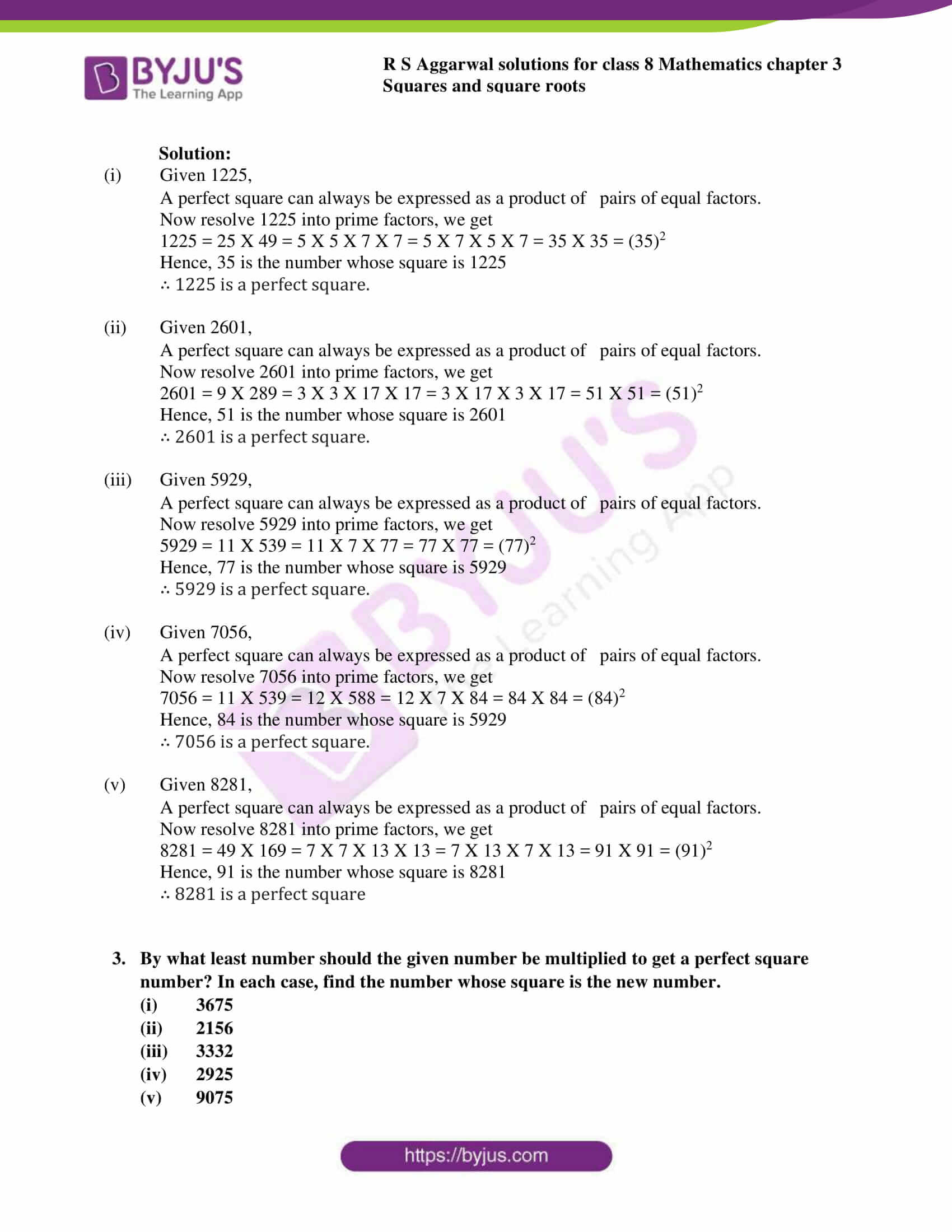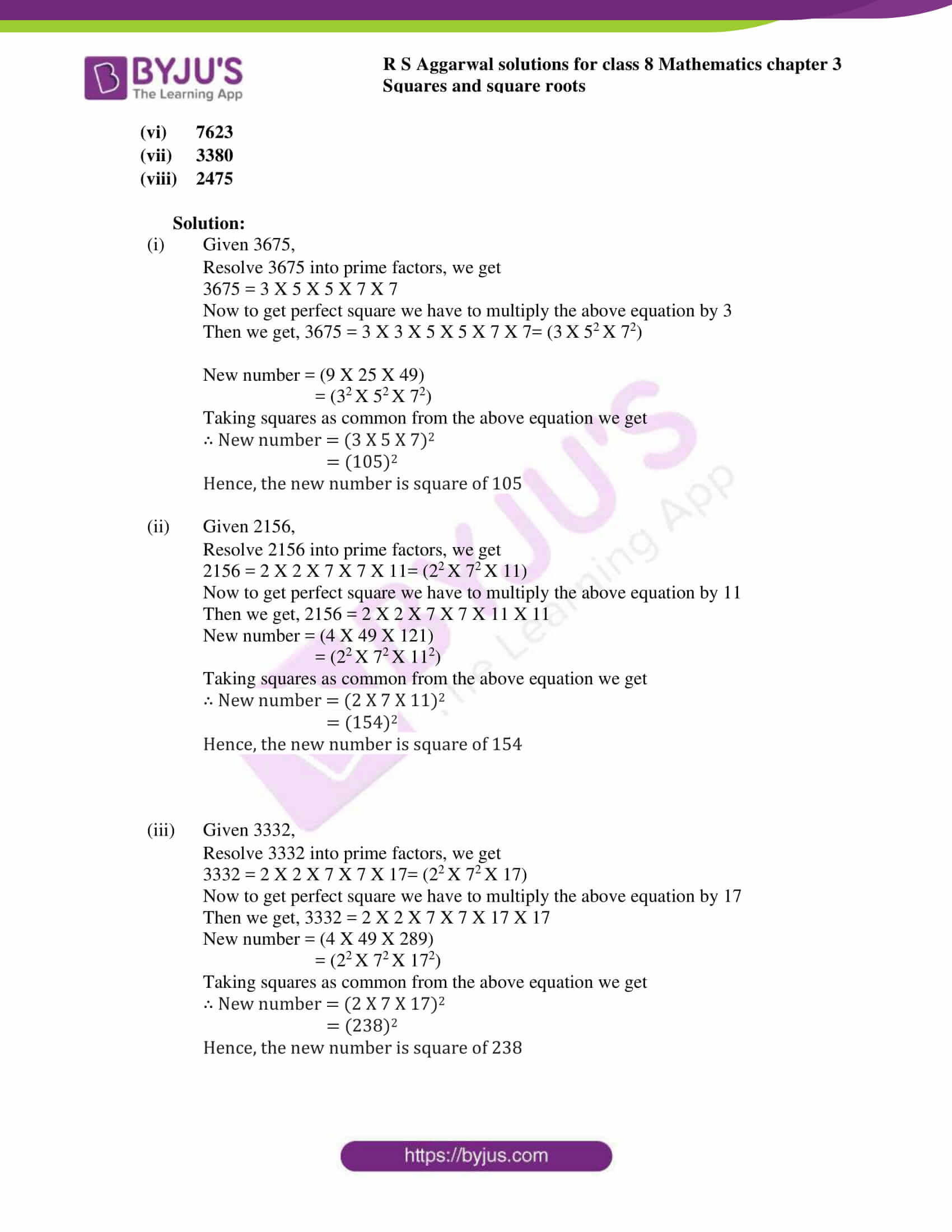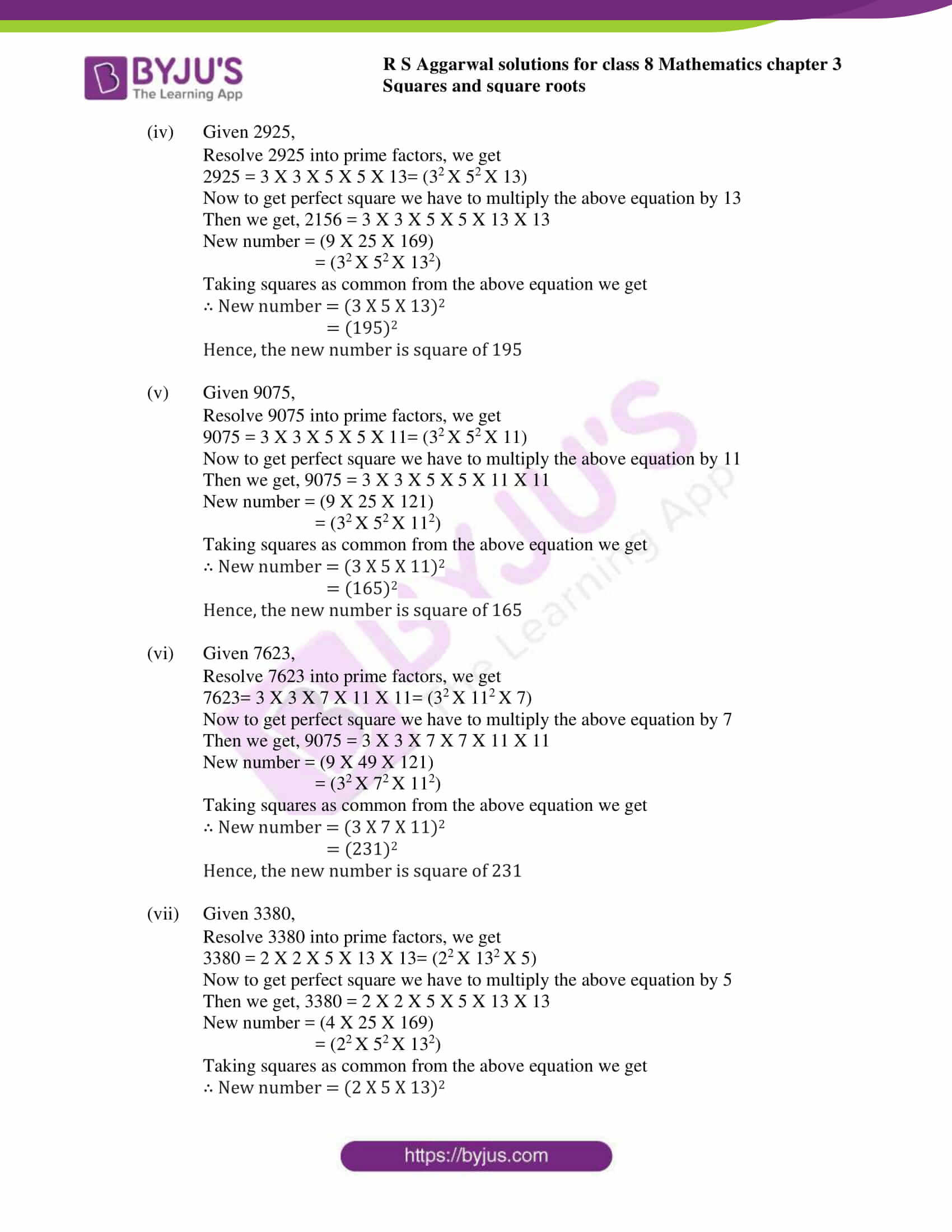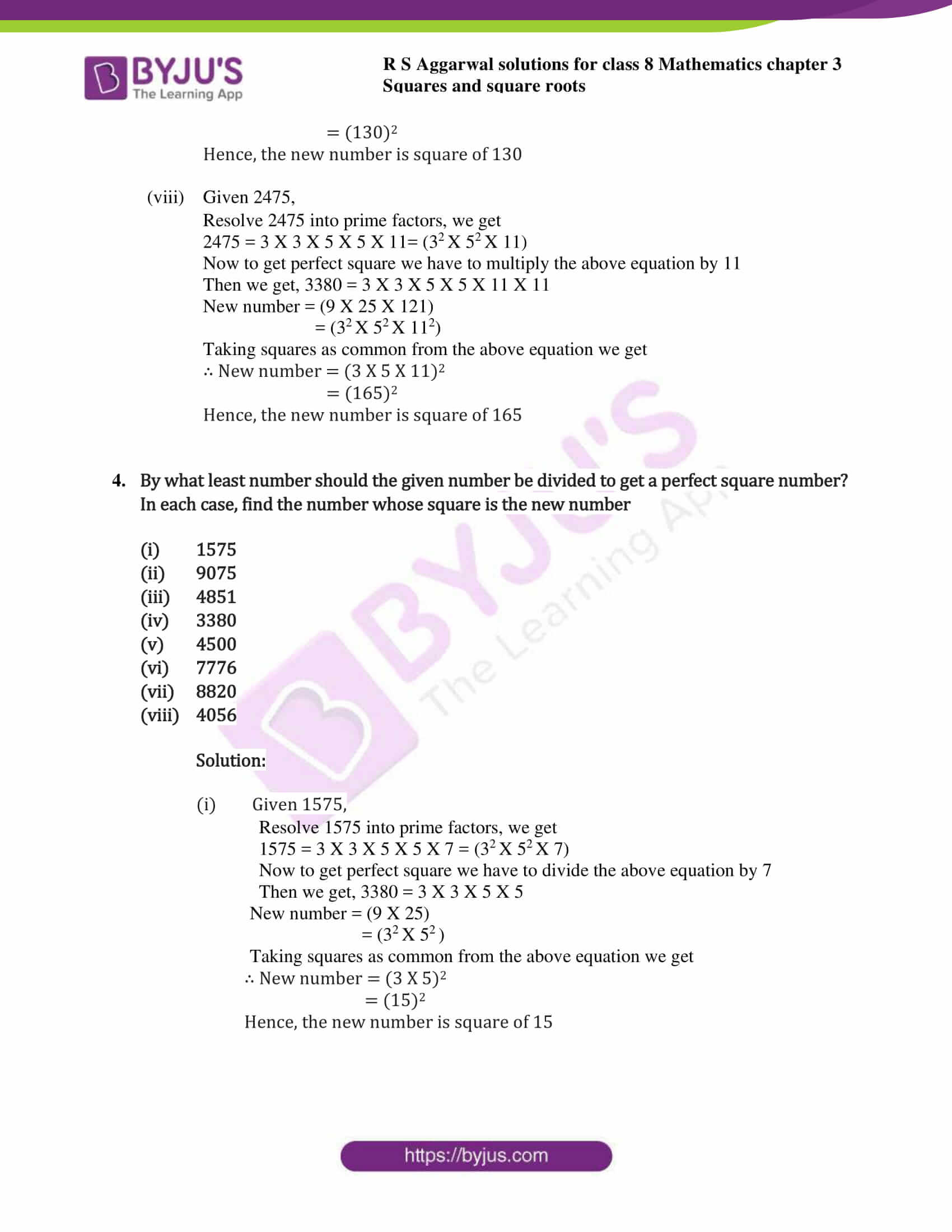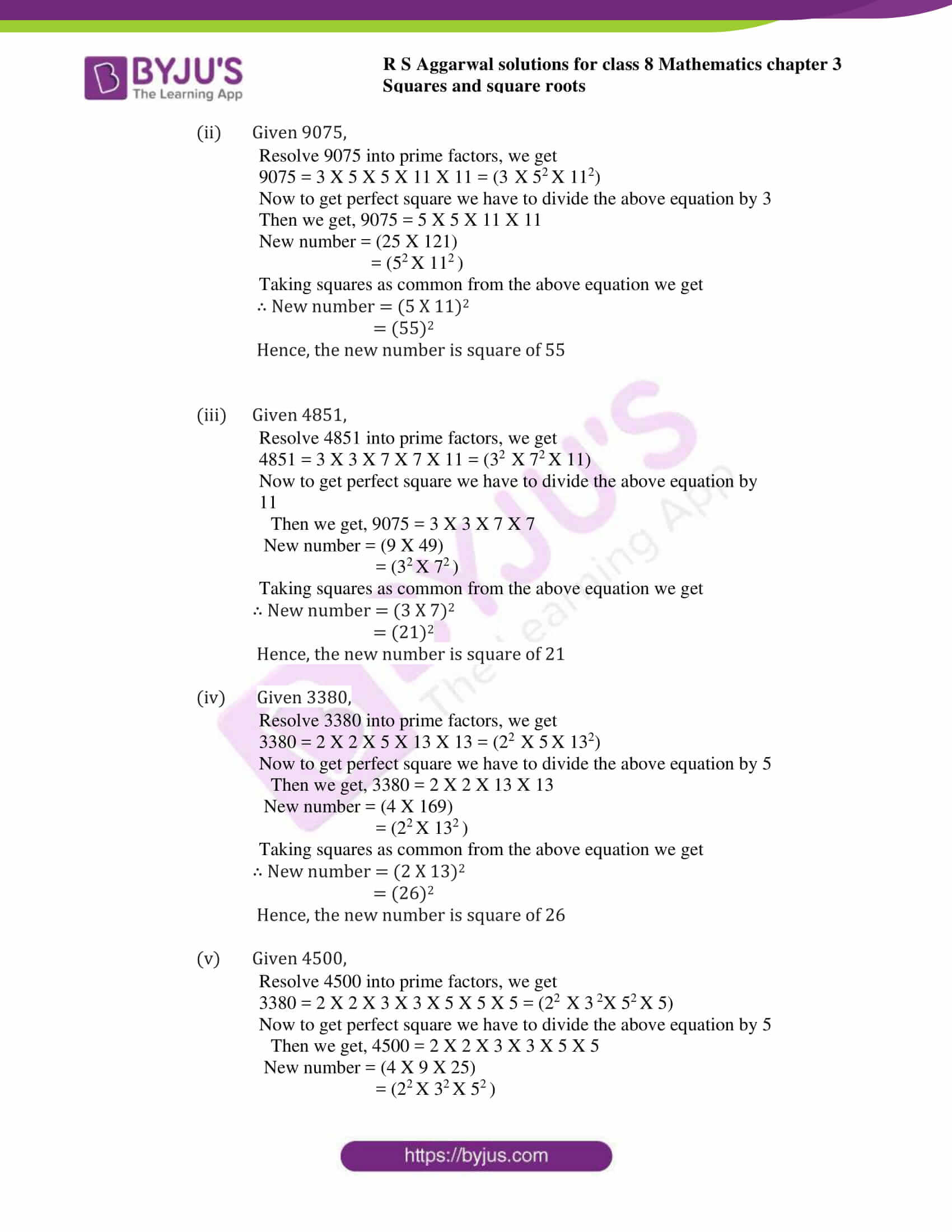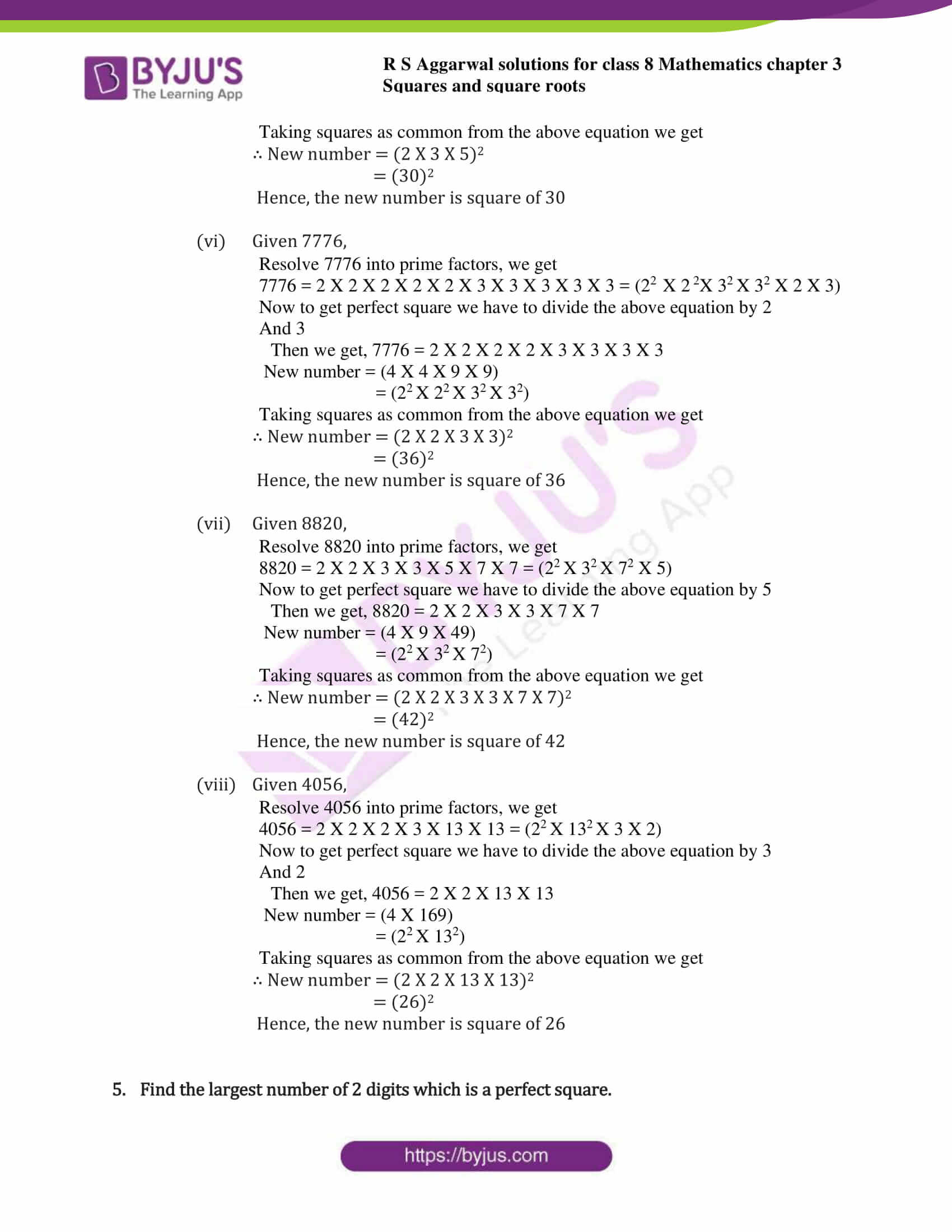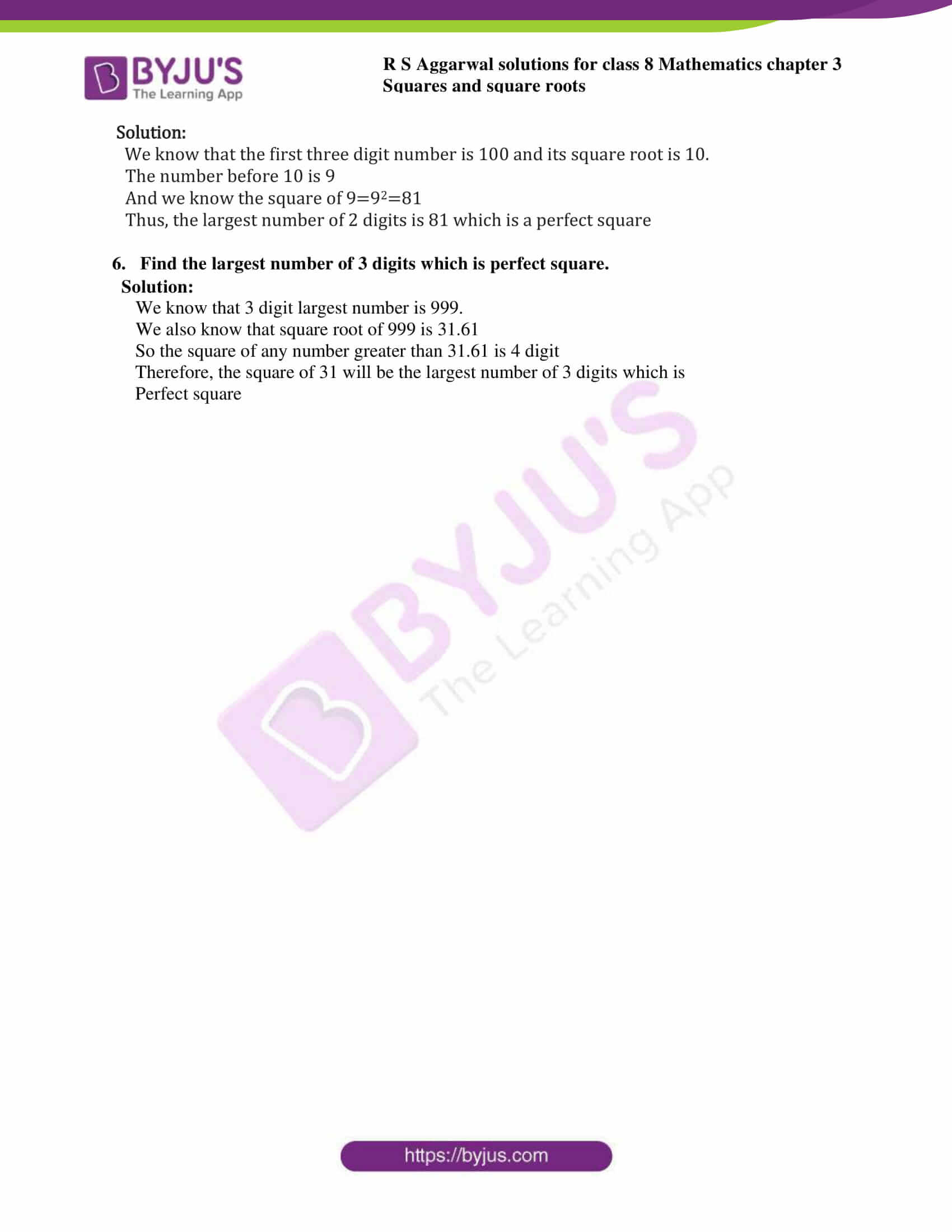### Access answers to Maths RS Aggarwal Solutions for Class 8 Chapter 3 – Squares and Square Roots Exercise 3A

Q1. Using the prime factorization method, find which of the following numbers are perfect squares:

(i) 441

(ii) 576

(iii) 11025

(iv) 1176

(v) 5625

(vi) 9075

(vii) 4225

(viii) 1089

Solution:

1. Given 441,

A perfect square can always be expressed as a product of pairs of equal factors.

Now resolve 441 into prime factors, we get

441 = 49 X 9 = 7 X 7 X 3 X 3 = 7 X 3 X 7 X 3 = 21 X 21 = (21)2

Hence, 21 is the number whose square is 441

∴ 441 is a perfect square.

2. Given 576

A perfect square can always be expressed as a product of pairs of equal factors.

Now resolve 576 into prime factors, we get

576 = 64 X 9 = 8 X 8 X 3 X 3 = 8 X 3 X 8 X 3 = 24 X 24 = (24)2

Hence, 24 is the number whose square is 576

∴ 576 is a perfect square.

3. Given 11025

A perfect square can always be expressed as a product of pairs of equal factors.

Now resolve 11025 into prime factors, we get

11025 = 441 X 25 = 49 X 9 = 7 X 7 X 3 X 3 X 5 X 5 = 7 X 3 X 5 X 7 X 3 X 5 = 105 X 105 = (105)2

Hence, 105 is the number whose square is 11025

∴ 11025 is a perfect square.

4. Given 1176

A perfect square can always be expressed as a product of pairs of equal factors.

Now resolve 1176 into prime factors, we get

1176 = 7 X 168

Again resolve 168 into prime factors we get,

1176 = 7 X 168 = 7 X 21 X 8 = 7 X 7 X 3 X 2 X 2 X 2

1176 cannot be expressed as a product of two numbers.

Thus 1176 is not a perfect square.

5. Given 5625

A perfect square can always be expressed as a product of pairs of equal factors.

Now resolve 5625 into prime factors, we get

5625 = 225 X 25 = 9 X 25 X 25 = 3 X 3 X 5 X 5 X 5 X 5 = 3 X 5 X 5 X 3 X 5 X 5

= 75 X 75 = (75)2

Hence, 75 is the number whose square is 5625

∴ 5625 is a perfect square.

6. Given 9075

A perfect square can always be expressed as a product of pairs of equal factors.

Now resolve 9075 into prime factors, we get

9075 = 25 X 363

Again resolve 363 into prime factors we get,

9075 = 25 X 363 = 25 X 121 X 3 = 5 X 5 X 11 X 11 X 3 =5 X 11 X 5 X 11 X 3 = 55 X 55 X 3

1176 cannot be expressed as a product of two numbers.

Thus 1176 is not a perfect square.

7. Given 4225

A perfect square can always be expressed as a product of pairs of equal factors.

Now resolve 4225 into prime factors, we get

4225 = 25 X 169 = 25 X 13 X 13 = 5 X 5 X 13 X 13 = 5 X 13 X 5 X 13

= 65 X 65 = (65)2

Hence, 65 is the number whose square is 4225

∴ 4225 is a perfect square.

8. Given 1089

A perfect square can always be expressed as a product of pairs of equal factors.

Now resolve 1089 into prime factors, we get

1089 = 9 X 121 = 3 X 3 X 11 X 11 = 3 X 11 X 3 X 11

= 33 X 33 = (33)2

Hence, 33 is the number whose square is 1089

∴ 1089 is a perfect square.

Q2. Show that each of the following numbers is a perfect square. In each case, find the number whose square is the given number:

(i) 1225

(ii) 2601

(iii) 5929

(iv) 7056

(v) 8281

Solution:

1. Given 1225

A perfect square can always be expressed as a product of pairs of equal factors.

Now resolve 1225 into prime factors, we get

1225 = 25 X 49 = 5 X 5 X 7 X 7 = 5 X 7 X 5 X 7 = 35 X 35 = (35)2

Hence, 35 is the number whose square is 1225

∴ 1225 is a perfect square.

2. Given 2601

A perfect square can always be expressed as a product of pairs of equal factors.

Now resolve 2601 into prime factors, we get

2601 = 9 X 289 = 3 X 3 X 17 X 17 = 3 X 17 X 3 X 17 = 51 X 51 = (51)2

Hence, 51 is the number whose square is 2601

∴ 2601 is a perfect square.

3. Given 5929

A perfect square can always be expressed as a product of pairs of equal factors.

Now resolve 5929 into prime factors, we get

5929 = 11 X 539 = 11 X 7 X 77 = 77 X 77 = (77)2

Hence, 77 is the number whose square is 5929

∴ 5929 is a perfect square.

4. Given 7056

A perfect square can always be expressed as a product of pairs of equal factors.

Now resolve 7056 into prime factors, we get

7056 = 11 X 539 = 12 X 588 = 12 X 7 X 84 = 84 X 84 = (84)2

Hence, 84 is the number whose square is 5929

∴ 7056 is a perfect square.

5. Given 8281

A perfect square can always be expressed as a product of pairs of equal factors.

Now resolve 8281 into prime factors, we get

8281 = 49 X 169 = 7 X 7 X 13 X 13 = 7 X 13 X 7 X 13 = 91 X 91 = (91)2

Hence, 91 is the number whose square is 8281

∴ 8281 is a perfect square

Q3. By what least number should the given number be multiplied to get a perfect square number? In each case, find the number whose square is the new number.

(i) 3675

(ii) 2156

(iii) 3332

(iv) 2925

(v) 9075

(vi) 7623

(vii) 3380

(viii) 2475

Solution:

1. Given 3675

Resolve 3675 into prime factors, we get

3675 = 3 X 5 X 5 X 7 X 7

Now to get perfect square we have to multiply the above equation by 3

Then we get, 3675 = 3 X 3 X 5 X 5 X 7 X 7= (3 X 52 X 72)

New number = (9 X 25 X 49)

= (32 X 52 X 72)

Taking squares as common from the above equation we get

∴ New number = (3 X 5 X 7)2

= (105)2

Hence, the new number is square of 105

2. Given 2156

Resolve 2156 into prime factors, we get

2156 = 2 X 2 X 7 X 7 X 11= (22 X 72 X 11)

Now to get perfect square we have to multiply the above equation by 11

Then we get, 2156 = 2 X 2 X 7 X 7 X 11 X 11

New number = (4 X 49 X 121)

= (22 X 72 X 112)

Taking squares as common from the above equation we get

∴ New number = (2 X 7 X 11)2

= (154)2

Hence, the new number is square of 154

3. Given 3332

Resolve 3332 into prime factors, we get

3332 = 2 X 2 X 7 X 7 X 17= (22 X 72 X 17)

Now to get perfect square we have to multiply the above equation by 17

Then we get, 3332 = 2 X 2 X 7 X 7 X 17 X 17

New number = (4 X 49 X 289)

= (22 X 72 X 172)

Taking squares as common from the above equation we get

∴ New number = (2 X 7 X 17)2

= (238)2

Hence, the new number is square of 238

4. Given 2925

Resolve 2925 into prime factors, we get

2925 = 3 X 3 X 5 X 5 X 13= (32 X 52 X 13)

Now to get perfect square we have to multiply the above equation by 13

Then we get, 2156 = 3 X 3 X 5 X 5 X 13 X 13

New number = (9 X 25 X 169)

= (32 X 52 X 132)

Taking squares as common from the above equation we get

∴ New number = (3 X 5 X 13)2

= (195)2

Hence, the new number is square of 195

5. Given 9075

Resolve 9075 into prime factors, we get

9075 = 3 X 3 X 5 X 5 X 11= (32 X 52 X 11)

Now to get perfect square we have to multiply the above equation by 11

Then we get, 9075 = 3 X 3 X 5 X 5 X 11 X 11

New number = (9 X 25 X 121)

= (32 X 52 X 112)

Taking squares as common from the above equation we get

∴ New number = (3 X 5 X 11)2

= (165)2

Hence, the new number is square of 165

6. Given 7623

Resolve 7623 into prime factors, we get

7623= 3 X 3 X 7 X 11 X 11= (32 X 112 X 7)

Now to get perfect square we have to multiply the above equation by 7

Then we get, 9075 = 3 X 3 X 7 X 7 X 11 X 11

New number = (9 X 49 X 121)

= (32 X 72 X 112)

Taking squares as common from the above equation we get

∴ New number = (3 X 7 X 11)2

= (231)2

Hence, the new number is square of 231

7. Given 3380

Resolve 3380 into prime factors, we get

3380 = 2 X 2 X 5 X 13 X 13= (22 X 132 X 5)

Now to get perfect square we have to multiply the above equation by 5

Then we get, 3380 = 2 X 2 X 5 X 5 X 13 X 13

New number = (4 X 25 X 169)

= (22 X 52 X 132)

Taking squares as common from the above equation we get

∴ New number = (2 X 5 X 13)2

= (130)2

Hence, the new number is square of 130

8. Given 2475

Resolve 2475 into prime factors, we get

2475 = 3 X 3 X 5 X 5 X 11= (32 X 52 X 11)

Now to get perfect square we have to multiply the above equation by 11

Then we get, 3380 = 3 X 3 X 5 X 5 X 11 X 11

New number = (9 X 25 X 121)

= (32 X 52 X 112)

Taking squares as common from the above equation we get

∴ New number = (3 X 5 X 11)2

= (165)2

Hence, the new number is square of 165

4. By what least number should the given number be divided to get a perfect square number? In each case, find the number whose square is the new number

(i) 1575

(ii) 9075

(iii) 4851

(iv) 3380

(v) 4500

(vi) 7776

(vii) 8820

(viii) 4056

Solution:

1. Given 1575

Resolve 1575 into prime factors, we get

1575 = 3 X 3 X 5 X 5 X 7 = (32 X 52 X 7)

Now to get perfect square we have to divide the above equation by 7

Then we get, 3380 = 3 X 3 X 5 X 5

New number = (9 X 25)

= (32 X 52 )

Taking squares as common from the above equation we get

∴ New number = (3 X 5)2

= (15)2

Hence, the new number is square of 15

2. Given 9075

Resolve 9075 into prime factors, we get

9075 = 3 X 5 X 5 X 11 X 11 = (3 X 52 X 112)

Now to get perfect square we have to divide the above equation by 3

Then we get, 9075 = 5 X 5 X 11 X 11

New number = (25 X 121)

= (52 X 112 )

Taking squares as common from the above equation we get

∴ New number = (5 X 11)2

= (55)2

Hence, the new number is square of 55

3. Given 4851

Resolve 4851 into prime factors, we get

4851 = 3 X 3 X 7 X 7 X 11 = (32 X 72 X 11)

Now to get perfect square we have to divide the above equation by

11

Then we get, 9075 = 3 X 3 X 7 X 7

New number = (9 X 49)

= (32 X 72 )

Taking squares as common from the above equation we get

∴ New number = (3 X 7)2

= (21)2

Hence, the new number is square of 21

4. Given 3380

Resolve 3380 into prime factors, we get

3380 = 2 X 2 X 5 X 13 X 13 = (22 X 5 X 132)

Now to get perfect square we have to divide the above equation by 5

Then we get, 3380 = 2 X 2 X 13 X 13

New number = (4 X 169)

= (22 X 132 )

Taking squares as common from the above equation we get

∴ New number = (2 X 13)2

= (26)2

Hence, the new number is square of 26

5. Given 4500

Resolve 4500 into prime factors, we get

3380 = 2 X 2 X 3 X 3 X 5 X 5 X 5 = (22 X 3 2X 52 X 5)

Now to get perfect square we have to divide the above equation by 5

Then we get, 4500 = 2 X 2 X 3 X 3 X 5 X 5

New number = (4 X 9 X 25)

= (22 X 32 X 52 )

Taking squares as common from the above equation we get

∴ New number = (2 X 3 X 5)2

= (30)2

Hence, the new number is square of 30

6. Given 7776

Resolve 7776 into prime factors, we get

7776 = 2 X 2 X 2 X 2 X 2 X 3 X 3 X 3 X 3 X 3 = (22 X 2 2X 32 X 32 X 2 X 3)

Now to get perfect square we have to divide the above equation by 2

And 3

Then we get, 7776 = 2 X 2 X 2 X 2 X 3 X 3 X 3 X 3

New number = (4 X 4 X 9 X 9)

= (22 X 22 X 32 X 32)

Taking squares as common from the above equation we get

∴ New number = (2 X 2 X 3 X 3)2

= (36)2

Hence, the new number is square of 36

7. Given 8820

Resolve 8820 into prime factors, we get

8820 = 2 X 2 X 3 X 3 X 5 X 7 X 7 = (22 X 32 X 72 X 5)

Now to get perfect square we have to divide the above equation by 5

Then we get, 8820 = 2 X 2 X 3 X 3 X 7 X 7

New number = (4 X 9 X 49)

= (22 X 32 X 72)

Taking squares as common from the above equation we get

∴ New number = (2 X 2 X 3 X 3 X 7 X 7)2

= (42)2

Hence, the new number is square of 42

8. Given 4056

Resolve 4056 into prime factors, we get

4056 = 2 X 2 X 2 X 3 X 13 X 13 = (22 X 132 X 3 X 2)

Now to get perfect square we have to divide the above equation by 3

And 2

Then we get, 4056 = 2 X 2 X 13 X 13

New number = (4 X 169)

= (22 X 132)

Taking squares as common from the above equation we get

∴ New number = (2 X 2 X 13 X 13)2

= (26)2

Hence, the new number is square of 26

5. Find the largest number of 2 digits which is a perfect square.

Solution:

We know that the first three digit number is 100 and its square root is 10.

The number before 10 is 9

And we know the square of 9=92=81

Thus, the largest number of 2 digits is 81 which is a perfect square

6. Find the largest number of 3 digits which is perfect square.

Solution:

We know that 3 digit largest number is 999.

We also know that square root of 999 is 31.61

So the square of any number greater than 31.61 is 4 digit

Therefore, the square of 31 will be the largest number of 3 digits which is

Perfect square

## RS Aggarwal Solutions for Class 8 Maths Chapter 3 – Squares and Square Roots Exercise 3A

Exercise 3A of RS Aggarwal Solutions for Chapter 3, Squares and Square Roots deals with the basic concepts related to a perfect square. We can say that this exercise mainly deals with the perfect squares or square numbers that the students have learned in this chapter. The first exercise of the chapter primarily deals with the fundamental explanations of properties perfect squares. Some of the topics focused prior to exercise 3A include the following.

• Definition of squares
• Examples of perfect squares
• Definition of perfect squares
• Finding the perfect square by prime factorisation method

The RS Aggarwal Solutions can help the students in practising and learning each and every concept as it provides solutions to all questions asked in the RS Aggarwal textbook. Those who aim to score high in the Maths of class 8 are advised to practice all the questions present in RS Aggarwal as many times as possible.